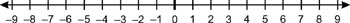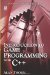# 2.3 Directed Numbers

## 2.3 Directed Numbers

So far, examples have only featured smaller numbers being subtracted from larger numbers, such as 53, 105, 71, etc. However, what will the result be if a larger number is taken from a smaller number, such as 35? Consider the following number line:Figure 2.1

Numbers to the left of 0 (numbers less than 0) are called negative numbers and will be the result when larger numbers are subtracted from smaller numbers. Numbers to the right of 0 are called positive numbers. To denote whether a number is positive or negative it is prefixed by a sign: for negative or + for positive. For example, 5 ("minus five") is negative while +3 ("plus three") is positive. The numbers encountered in previous sections have no sign; when this is the case, they are assumed to be positive. In other words, we do not need to prefix positive numbers with a + sign, but negative numbers need to be prefixed with a sign.

• 5 (minus is the sign and 5 is the absolute value)

### 2.3.1 Addition with Negatives

Adding positive numbers results in an answer that is larger, and adding negative numbers results in an answer that is smaller. Adding a negative term can be considered as subtraction. You can use the number line to count from left to right the effect a negative number has when added. Here are some examples:

 5 + 5 = 10 (no negatives here) 5 + (−3) = 2 This is the same as 5 − 3. 10 + (−5) = 5 This is the same as 10 − 5. 2 + (−5) = − 3 This is the same as 2 − 5. − 2 + (−3) = − 5 This is the same as −2 − 3.

In short, if the signs are the same, such as (5 + 5) or (2 3), then the terms should be added and the sign remains the same. If the signs are different, subtract the smaller number from the larger and the sign will be that of the larger number.

### 2.3.2 Subtraction with Negatives

Subtracting a negative number means to add. 5 (5) = 10, which means5 + 5 = 10. Also: 5 (5) = 5 + 5 = 0.

Again, if the signs are the same, such as (5 + 5), then the terms should be added and the sign remains the same. If the signs are different, subtract the smaller number from the larger and the sign will be that of the larger number.

### 2.3.3 Multiplication with Negatives

When two factors are multiplied, the result will be a positive answer if their signs are the same. If their signs are different, the result will be a negative answer.

In short, multiply two factors as before, ignoring the signs. After multiplying, the sign should be selected: negative for factors with different signs and positive for those with the same sign.

 −2 × 5 = −10 2 × 5 = 10 2 × −5 = −10 −2 × −5 = 10

### 2.3.4 Division with Negatives

As with multiplication, if the signs of both the dividend and divisor are the same, the quotient will be positive; otherwise it will be negative.

 −2 / −8 = 1/4 −4 / 2 = −2 8 / 2 = 4Introduction to Game Programming with C++ (Wordware Game Developers Library)
ISBN: 1598220322
EAN: 2147483647
Year: 2007
Pages: 225
Authors: Alan Thorn

Similar book on Amazon

flylib.com © 2008-2017.
If you may any questions please contact us: flylib@qtcs.net